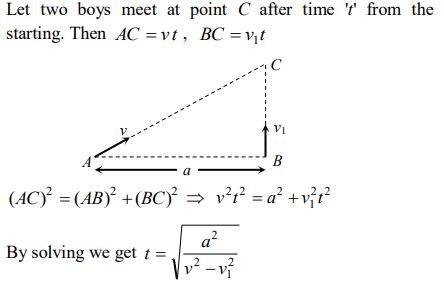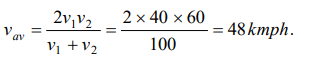## Motion in a Straight Line Questions and Answers Part-3

1. A person travels along a straight road for the first half time with a velocity $V_{1}$ and the next half time with a velocity $V_{2}$ . The mean velocity V of the man is
a) $\frac{2}{V}=\frac{1}{V_{1}}+\frac{1}{V_{2}}$
b) $V=\frac{V_{1}+V_{2}}{2}$
c) $V=\sqrt{V_{1}V_{2}}$
d) $V=\sqrt{\frac{V_{1}}{V_{2}}}$

Explanation: $V=\frac{V_{1}+V_{2}}{2}$

2. If a car covers 2/5th of the total distance with $V_{1}$ speed and 3/5th distance with $V_{2}$ then average speed is
a) $\frac{1}{2}\sqrt{V_{1}V_{2}}$
b) $\frac{V_{1}+V_{2}}{2}$
c) $\frac{2V_{1}V_{2}}{V_{1}+V_{2}}$
d) $\frac{5V_{1}V_{2}}{3V_{1}+2V_{2}}$

Explanation:3. The numerical ratio of displacement to the distance covered is always
a) Less than one
b) Equal to one
c) Equal to or less than one
d) Equal to or greater than one

Explanation: Since displacement is always less than or equal to distance, but never greater than distance. Hence numerical ratio of displacement to the distance covered is always equal to or less than one

4. A 100 m long train is moving with a uniform velocity of 45 km/hr. The time taken by the train to cross a bridge of length 1 km is
a) 58 s
b) 68 s
c) 78 s
d) 88 s

Explanation:5. A particle moves for 20 seconds with velocity 3 m/s and then velocity 4 m/s for another 20 seconds and finally moves with velocity 5 m/s for next 20 seconds. What is the average velocity of the particle
a) 3 m/s
b) 4 m/s
c) 5 m/s
d) Zero

Explanation:6. The correct statement from the following is
a) A body having zero velocity will not necessarily have zero acceleration
b) A body having zero velocity will necessarily have zero acceleration
c) A body having uniform speed can have only uniform acceleration
d) A body having non-uniform velocity will have zero acceleration

Explanation: When the body is projected vertically upward then at the highest point its velocity is zero but acceleration is not equal to zero ( g = 9.8 m/s2)

7. A bullet fired into a fixed target loses half of its velocity after penetrating 3 cm. How much further it will penetrate before coming to rest assuming that it faces constant resistance to motion?
a) 1.5 cm
b) 1.0 cm
c) 3.0 cm
d) 2.0 cm

Explanation:8. Two boys are standing at the ends A and B of a ground where $AB=a$  . The boy at B starts running in a direction perpendicular to AB with velocity $V_{1}$ . The boy at A starts running simultaneously with velocity v and catches the other boy in a time t, where t is
a) $a\diagup\sqrt{V^{2} + V_1^2}$
b) $\sqrt{a^{2}\diagup\left(V^{2} - V_1^2\right)}$
c) $a\diagup\left(V-V_1\right)$
d) $a\diagup\left(V+V_1\right)$

Explanation:9. A car travels half the distance with constant velocity of 40 kmph and the remaining half with a constant velocity of 60 kmph. The average velocity of the car in kmph is
a) 40
b) 45
c) 48
d) 5010. A particle experiences a constant acceleration for 20 sec after starting from rest. If it travels a distance $S_1$ in the first 10 sec and a distance $S_2$ in the next 10 sec, then
a) $S_{1}=S_{2}$
b) $S_{1}=S_{2}\diagup 3$
c) $S_{1}=S_{2}\diagup 2$
d) $S_{1}=S_{2}\diagup 4$